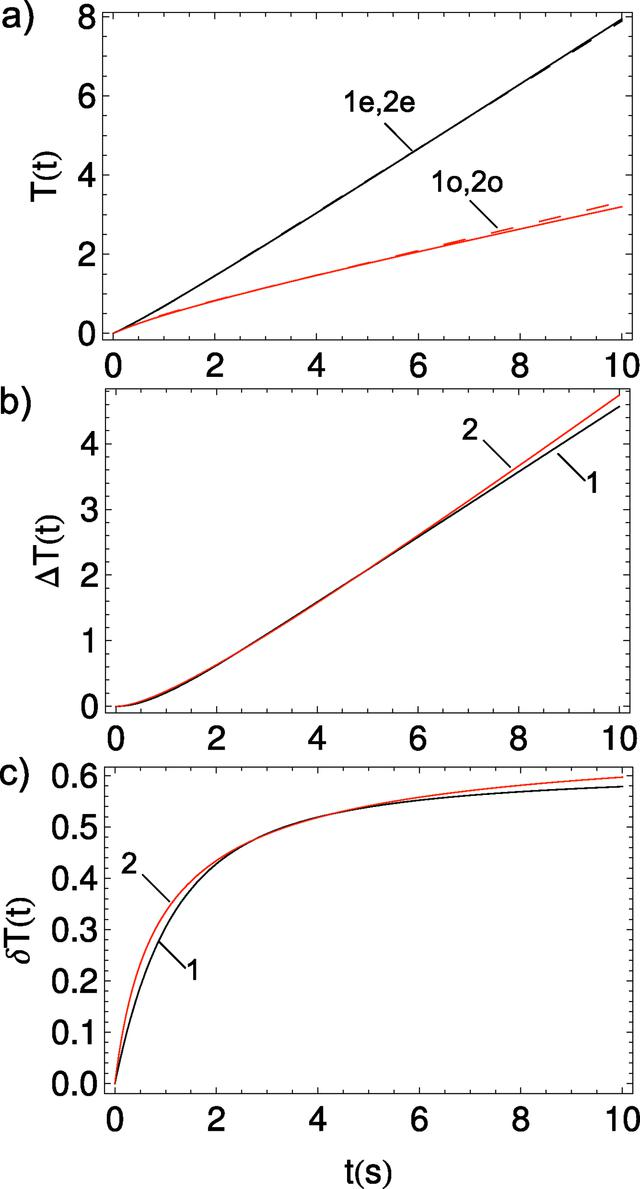disable zoom     view article Figure 11 Comparison of T(t) for original (index `o') and extended (index `e') Wang methods. (a) For two A(t) approximation models: exponential (1, black dashed) and hyperbolic (2, black solid). (b) Difference between the extended and original methods. (c) Relative difference when (b) is divided by the extended T(t) Wang method. In both (b) and (c) the exponential A(t) model is (1, black) and the hyperbolic A(t) model is (2, red).JOURNAL OFSYNCHROTRONRADIATION
ISSN: 1600-5775
Volume 25| Part 3| May 2018| Pages 833-847